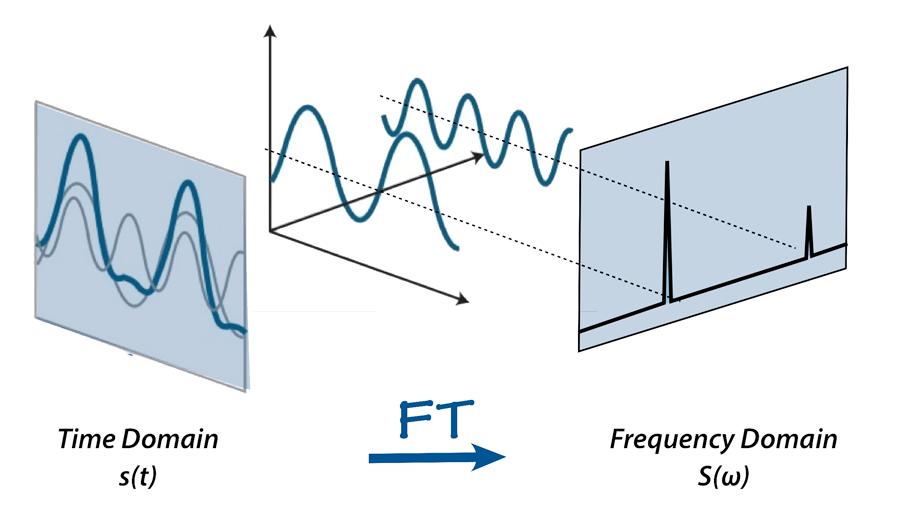### Fourier Transforms, Fraud, and Fun

I recently backwards engineered the algorithm that some unsavory characters were using to brute force find real credit card numbers and fraudulently charge them. The backbone to reverse engineering their algorithm was the Fourier Transform. I think that this is probably one of the most useful mathematical concepts that I ever learned. It falls right behind regressions, ANOVAs, and t-tests. If you were paying attention those were all statistical methods; Fourier transforms, however, are not statistical in the usual sense. You do need data to operate on, but I would actually dump this algorithm, usually firmly in the camp of feature engineering, although technically, it is a useful concept outside of data science in the wider world of mathematics and engineering.

At any rate, I thought that it would be useful to describe the problem that I faced and how to use a fourier transform to solve the problem. The fraudsters were basically picking cards just to see if they were active, real cards. They just seemed to be making up those card numbers. Now, the question was which card numbers do they pick next? So since card numbers are just 16 digit numbers I sorted the card numbers. Then I differenced them to see what increments they were going up by. Plotting these differences on a histogram, it became excruciatingly clear that the fraudsters were taking great pains to avoid going up by some multiple of 10. So for example, they avoided going up by 10, 20, and 30 card numbers, preferring instead to go up by numbers that were offset from these round numbers.

That was my first indication that these fellows were using some sort of algorithm to guess card numbers. They weren’t just picking random numbers. That’s when it occurred to me, that if they have an algorithm with some sort of periodicity to it, then a fourier transformation should in theory be able to pick up on the cycles, and potentially predict the card numbers that they would try next.

At this point, I think that it might be beneficial to see how a Fourier transform works.

### Moving to a Frequency Domain

Fourier transformations work by using a specific formula that will move you from a “time” domain to a frequency domain. The way that it does that, is that it combines sine waves of different frequencies at different amplitudes. This super-position of sine waves can actually be used to approximate any function, so long as you include enough frequencies. In fact, you can map out any arbitrary function with an infinite number of sine waves. So if you mark the amplitude at different frequencies, you can in effect, model the same data in a frequency domain. This principle comes in very handy for solving partial differential equations because you can get an exact solution analytically. But that isn’t the point. Once you have the frequency domain in place, you can in effect move forward in time according to the rules set down to calculate the Fourier transform in the first place.

To get a better idea, I think the following picture is really helpful. You have some function which you decompose into a bunch of sine waves of different frequencies. You then read off the amplitude coefficients and mark them on a graph with the frequencies on the x-axis and the amplitudes on the y-axis.If you reverse the process that you used to generate the fourier transform, the so-called (and aptly named) inverse fourier transform, you will recover the function that generated your data in the first place. That is what I did with the card numbers. When I took the fourier transform of the differenced card numbers, I got a graph with spikes of similar amplitudes at regular intervals. That’s when I knew that I had something special.

There was some cleaning up afterwards. Make sure that the card numbers that I generated were valid 16 digit card numbers by dealing with decimals, making sure the card numbers monotonically increased as in my sample, etc.

When all was said and done, I generated a list of 100 card numbers. The first couple were tried by the fraudsters within a few hours. So I knew at that point that I had their algorithm in my hands. From there it was relatively simple to stop the bad behavior.

If you have a problem like this that has you stumped, give me a call today at (801) 815-2922. I’d be more than happy to help you out.